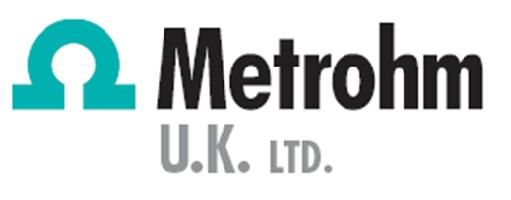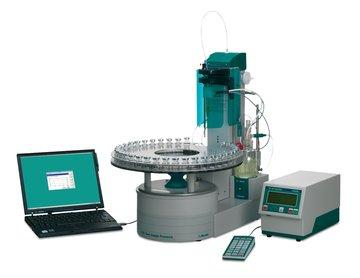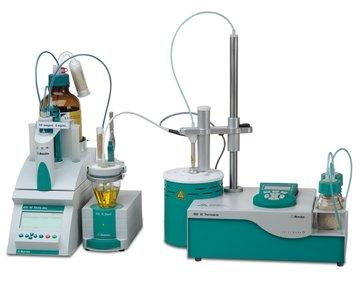## Microsoft word - basic theory_karl fischer titrationKarl Fischer Titration

What is a Karl Fischer chemical reaction?

I2 + 2H2 O+ SO2 -> 2HI + H2SO4
This reaction taking place in the presence of a base and a solvent. (a typical solvent could be methanol, and a base imidazole)
How does the Karl Fischer determination work?

A chemical reaction takes place between Iodine (I) and water (W) with the
reactants being in a 1 to 1 ratio between (I) & (W).
1x (I) + 1x (W) -> Reaction
Once the iodine in the Karl Fischer reagent is determined, the unknown concentration of water in the sample can be determined. I.e. as 1x(I) reacts with 1x(W), the amount of (I) used during the titration must be
equal to the unknown amount of water present in the sample.
Volumetric or Coulometric determination methods?

The Volumetric method is used for ppm levels up to 100% water i.e. "high level
moisture"
The Coulometric method is used for moisture levels in the range of 10 micrograms to 10mgs of water in a sample i.e. “low level moisture"
Coulometric Karl Fischer

With a coulometric Karl Fischer titration, the amount of water present is determined
by measuring the amount of current generated during the titration (Coulombs)
Coulombs are a measurement of current (amps) multiplied by the titration time in
seconds.
There is a relationship between the iodine (I) used in the titration, the samples water content (W) and the current.
Current (A) x time (secs) = Coulombs (C) ---> I + W ---> KF REACTION

2 x 96,485 Coulombs are needed to generate 1 mole of iodine and this iodine subsequently reacts 1 to 1 with the water in the KF reaction. How does a Coulometric titration work?
Coulometric KF titration's are preferable carried out within the pH RANGE 4-7
Ø Stage 1: Iodine generation
Instead of dispensing KF reagent as in Volumetric KF titration, the Metrohm KF instrumentation actually generates the reagent inside the reaction cell. A current flows through the reagent generating iodine at the anode electrode. Ø Stage 2: The Metrohm instrument detects the end of the titration (end-
Ø Stage 3: The Metrohm instrument subsequently calculates the moisture

Components of a Coulometric Titrator

The electrode system ….this consists of an anode and cathode platinum electrodes
which conducts current through the cell, generates iodine at the anode, which then
subsequently reacts with the water in the sample.
The platinum indicating electrode determines when & if iodine generation is necessary to react with any moisture in the sample. It does this by voltametrically sensing the presence of water and then continues to generate iodine until all the water in the sample has been reacted with….the KF end-point. From this titration the on board microprocessor in the Metrohm instrument calculates the total amount of current consumed in completing the titration and the time to completion in seconds. Based on the relationship between coulombs and iodine the exact amount of iodine generated is determined. Since water reacts in the 1:1 ratio with iodine, the amount of water can be calculated.
Volumetric Karl Fischer
How does volumetric Karl Fischer Titration work?

Volumetric KF determinations are preferentially carried out between pH 4-7
In volumetric KF titration the Metrohm KF Titrator controls 3 steps:
Ø It dispenses the KF reagent into the reaction cell. Ø It detects the end point of the reaction between the KF reagent and Ø It calculates the moisture content in the sample.# Components of a Metrohm Volumetric Titration:Ø Exchange unit (burette), this part of the system dispenses the KF reagent, which contains iodine, for reaction with the water present in the Ø The platinum measuring indicator electrode, in this set up, determines whether or not iodine needs to be added to react with any water present i.e. as before the water is detected voltametrically and as long as the electrode detects water, it will electronically instruct the burette to keep adding reagent. When all the water has reacted the burette stops dispensing and the end point is reached. Ø The onboard microprocessor records the volume of KF reagent dispensed, then based on the concentration of iodine in the KF reagent, it calculates how much water was present in the sample.

Karl Fischer Moisture Determination in Difficult Samples
For water content determinations using the Karl Fischer method on solids that are
either insoluble, react with the Karl Fischer reagent or for
other reasons cannot be transferred to the titration vessel
directly for either Coulometric or Volumetric
analysis…Metrohm offers KF Ovens.
These KF Ovens drive off thermally the moisture in the
samples and transfer it to the KF reaction vessel by means of
and inert carrier gas.

Automatic Oven Sample Processing
Metrohm offer a unique system for the automation of up to 35 samples, for either
volumetric or coulometric determination, with a new oven for
each sample . . . THE 874 OVEN SAMPLE PROCESSOR
Samples are placed in vials with rubber seals, which are then inserted into a rack. Stainless Steel needles pierce the rubber seal to introduce and remove a carrier gas; at the same time the vial is pressed into the hot oven. Dry air or nitrogen is led through the sample and the gas transfers the released water quantitatively to the titration cell contained in the sample changer. The moisture can now be determined easily by volumetric or coulometric KF titration, without any sample preparation, in less than 5 minutes / sample, plus there is a considerable reduction in KF reagent savings. Quick, Simple and allows you to save a lot of money! The full range of Karl Fischer Titration products can be viewed at